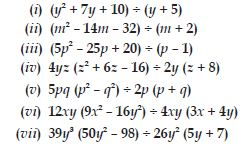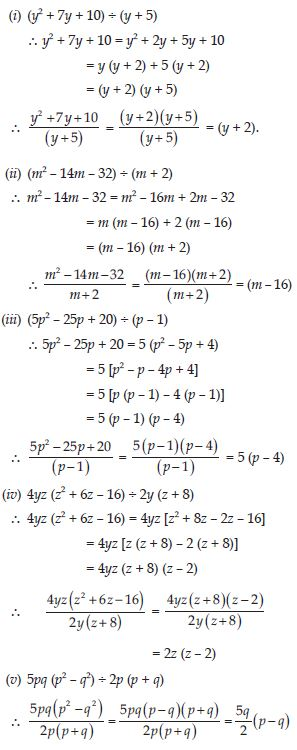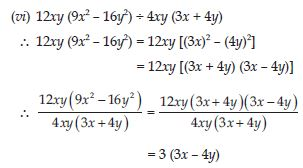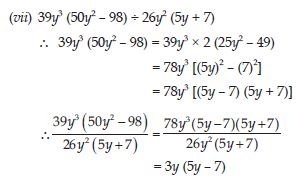# NCERT Solutions for Class 8 Math Chapter 14 - Factorisation

##### Question 1:

Factorise:
12x + 36

12x + 36 = 12 (x + 3)

##### Question 2:

Factorise:
22y - 33z

22y - 33z = 11 (2y - 3z)

##### Question 3:

Factorise:
14pq + 35pqr

14pq + 35pqr = 7pq (2 + 5r)

##### Question 4:

Find the common factors of the given terms:
12x, 36

12x, 36
12x = 2 × 2 × 3 × x
36 = 2 × 2 × 3 × 3
$\therefore$ The common factors are 2, 2, 3 and the highest
common factor is 12.

##### Question 5:

Find the common factors of the given terms:
2y, 22xy

2y, 22xy
2y = 2 × y
22xy = 2 × 11 × x × y
$\therefore$ The common factors are 2, y and the highest common factor is 2y.

##### Question 6:

Find the common factors of the given terms:
14pq, 28p2q2

14pq, 28p2q2
14pq = 2 × 7 × p × q
28p2q2 = 2 × 2 × 7 × p × p × q × q
$\therefore$ The common factors are 2, 7, p, q and the highest common factor is 14pq

##### Question 7:

Find the common factors of the given terms:
2x, 3x2, 4

2x, 3x2, 4
2x = 2 × x
3x2 = 3 × x × x
4 = 2 × 2
$\therefore$ The common factor is 1.

##### Question 8:

Find the common factors of the given terms:
6abc, 24ab2, 12a2b

6abc, 24ab2, 12a2b
6abc = 2 × 3 × a × b × c
24ab2 = 2 × 2 × 2 × 3 × a × b × b
12a2b = 2 × 2 × 3 × a × a × b
$\therefore$ The common factors are 2, 3, a, b and the
highest common factor is 6ab.

##### Question 9:

Find the common factors of the given terms:
16x3b, - 4x2, 32x

16x3b, - 4x2b, 32x
16x3 = 2 × 2 × 2 × 2 × x × x × x
- 4x2 = - 1 × 2 × 2 × x × x
32x = 2 × 2 × 2 × 2 × 2 × x
$\therefore$ The common factors are 2, 2, x and the highest common factor is 4x.

##### Question 10:

Find the common factors of the given terms:
10pq, 20qr, 30 rp

10pq, 20qr, 30rp
10pq = 2 × 5 × p × q
20qr = 2 × 2 × 5 × q × r
30rp = 2 × 3 × 5 × r × p
$\therefore$ The common factors are 2, 5 and the highest
common factor is 10

##### Question 11:

Find the common factors of the given terms:
3x2y3, 10x3y2, 6x2y2z

3x2y3, 10x3y2, 6x2y2z
3x2y3 = 3 × x × x × y × y × y
10x3y2 = 2 × 5 × x × x × x × y × y
6x2y2z = 2 × 3 × x × x × y × y × z
$\therefore$ The common factors are x, x, y, y and the highest common factor is x2y2.

##### Question 12:

Factorise the following expressions:
7x - 42

7x - 42 = 7 (x - 6)

##### Question 13:

Factorise the following expressions:
6p - 12q

6p - 12q = 6 (p - 2q)

##### Question 14:

Factorise the following expression: 7a2 + 14a

7a2 + 14a = 7a (a + 2)

##### Question 15:

Factorise the following expression: -16z + 20z3

- 16z + 20z3 = -4z (4 - 5z2)

##### Question 16:

Factorise the following expression: 20l2m + 30alm

20l2m + 30alm = 10lm (2l + 3a)

##### Question 17:

Factorise the following expression: 5x2y - 15xy2

5x2y - 15xy2 = 5xy (x - 3y)

##### Question 18:

Factorise the following expression: 10a2 - 15b2 + 20c2

10a2 - 15b2 + 20c2 = 5 (2a2 - 3b2 + 4c2)

##### Question 19:

Factorise the following expression: - 4a2 + 4ab - 4ca

- 4a2 + 4ab - 4ca = - 4a (a - b + c)

##### Question 20:

Factorise the following expression: x2yz + xy2z + xyz2

x2yz + xy2z + xyz2 = xyz (x + y + z)

##### Question 21:

Factorise the following expression: ax2y + bxy2 + cxyz

ax2y + bxy2 + cxyz = xy (ax + by + cz)

##### Question 22:

Factorise:
x2 + xy + 8x + 8y

x2 + xy + 8x + 8y
= x (x + y) + 8 (x + y)
= (x + y) (x + 8)

##### Question 23:

Factorise:
15xy - 6x + 5y - 2

15xy - 6x + 5y - 2
= 3x (5y - 2) + 1 (5y - 2)
= (5y - 2) (3x + 1)

##### Question 24:

Factorise:
ax + bx - ay - by

ax + bx - ay - by
= x (a + b) - y (a + b)
= (a + b) (x - y)

##### Question 25:

Factorise:
15pq + 15 + 9q + 25p

15pq + 15 + 9q + 25p
= 15pq + 25p + 9q + 15
= 5p (3q + 5) + 3 (3q + 5)
= (3q + 5) (5p + 3)

##### Question 26:

Factorise:
z - 7 + 7xy - xyz

z - 7 + 7xy - xyz
= z - xyz - 7 + 7xy
= z (1 - xy) - 7 (1 - xy)
= (1 - xy) (z - 7)

##### Question 27: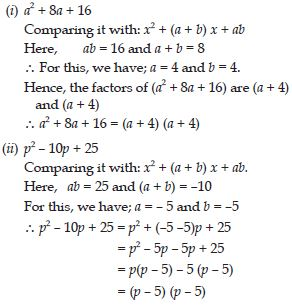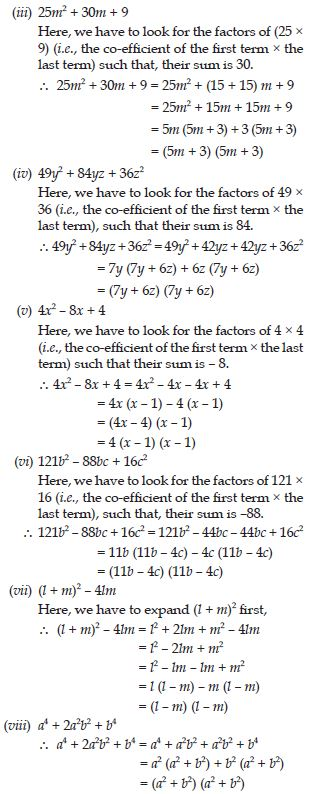##### Question 28:

Factorise:
4p2 - 9q2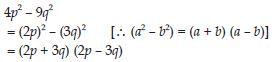##### Question 29:

Factorise:
63a2 - 112b2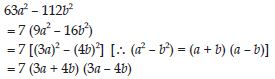##### Question 30:

Factorise:
49x2 - 36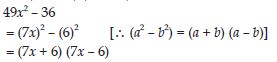##### Question 31:

Factorise:
16x5 - 144x3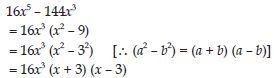##### Question 32:

Factorise:
(l + m)2 - (l - m)2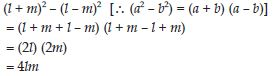##### Question 33:

9x2y2 - 16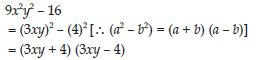##### Question 34:

(x2 - 2xy + y2) - z2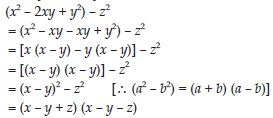##### Question 35:

25a2 - 4b2 + 28bc - 49c2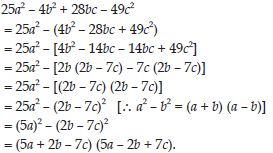##### Question 36: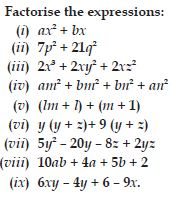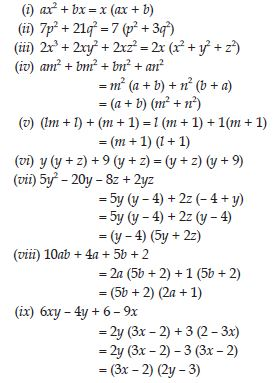##### Question 37: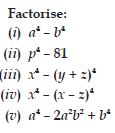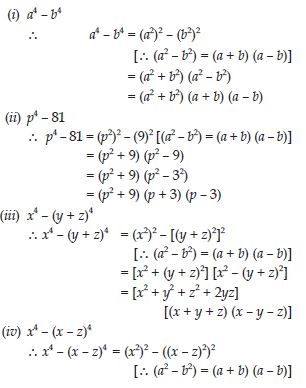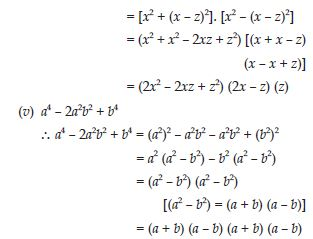##### Question 38:

Factorise the following expressions: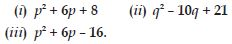##### Question 39: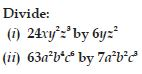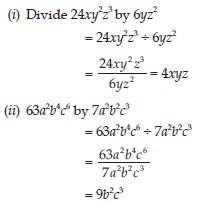##### Question 40: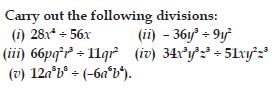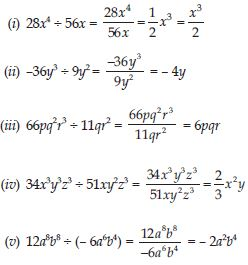##### Question 41: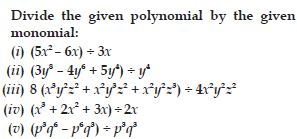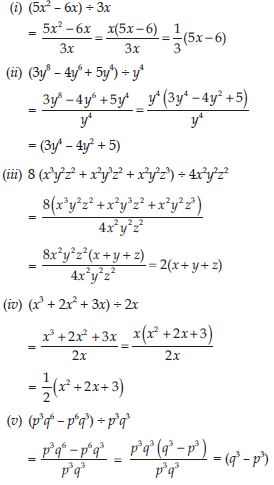##### Question 42:

Work out the following divisions:
(i) (10x - 25) $÷$ 5
(ii) (10x - 25) $÷$ (2x - 5)
(iii) 10y (6y + 21) $÷$ 5 (2y + 7)
(iv) 9x2y2 (3z - 24) $÷$ 27xy (z - 8)
(v) 96abc (3a - 12) (5b - 30) $÷$ 144 (a - 4) (b - 6).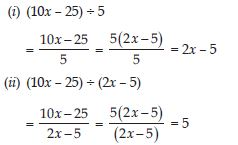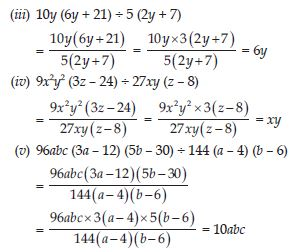##### Question 43:

Divide as directed:
(i) 5 (2x + 1) (3x + 5) $÷$ (2x + 1)
(ii) 26xy (x + 5) (y - 4) $÷$ 13x (y - 4)
(iii) 52pqr (p + q) (q + r) (r + p) $÷$ 104pq (q + r)(r + p)
(iv) 20 (y + 4) (y2 + 5y + 3) $÷$ 5(y + 4)
(v) x (x + 1) (x + 2) (x + 3) $÷$ x (x + 1).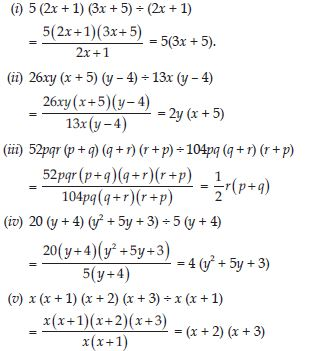##### Question 44:

Factorise the expressions and divide them as directed: